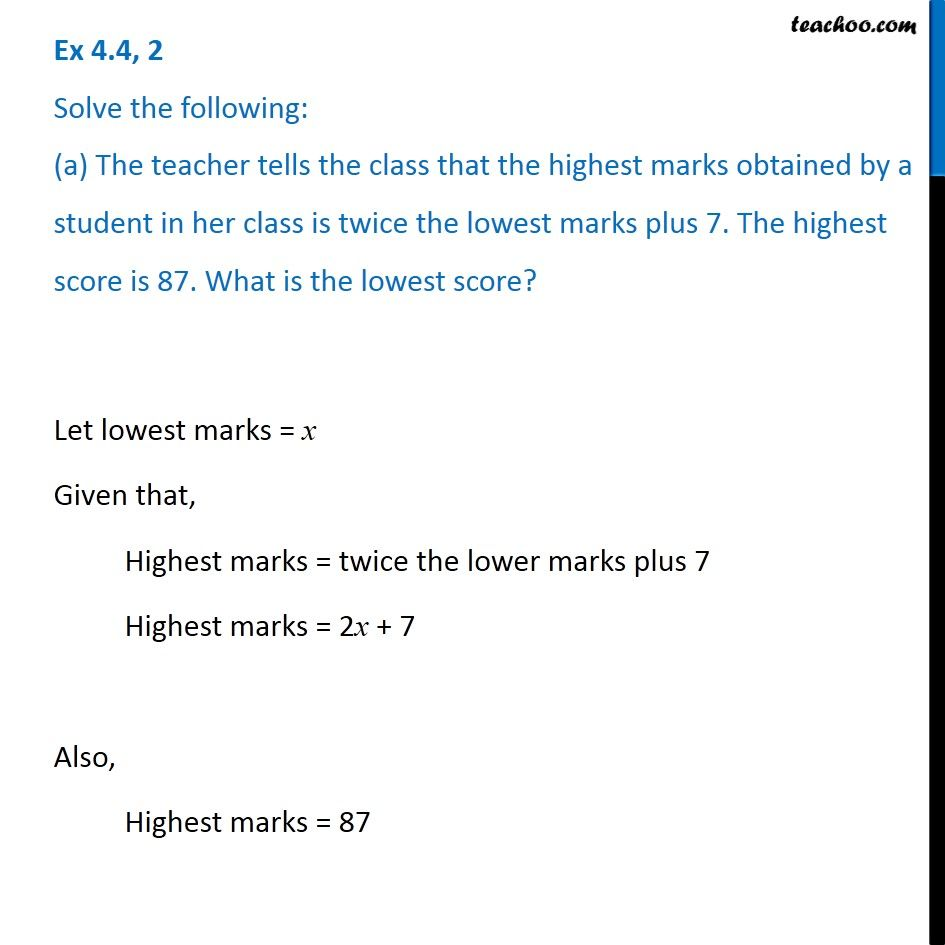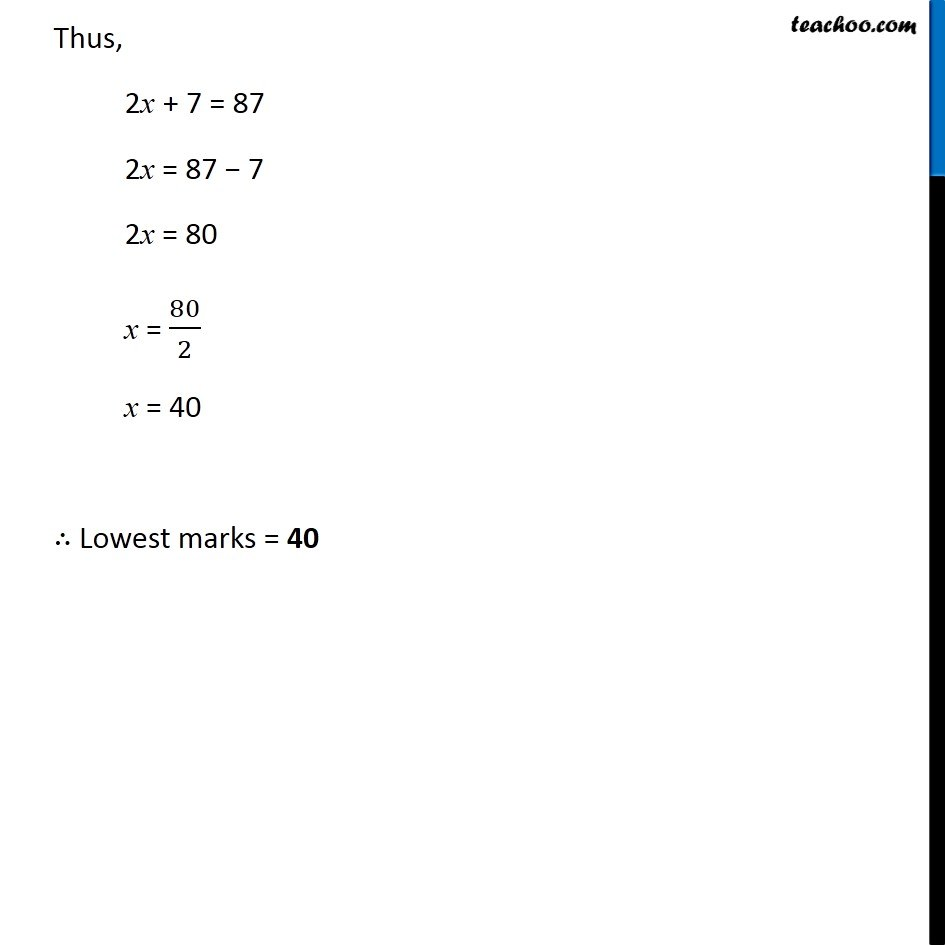Making equations from statements and solving

Chapter 4 Class 7 Simple Equations
Concept wiseIntroducing your new favourite teacher - Teachoo Black, at only ₹83 per month

### Transcript

Ex 4.4, 2 Solve the following: (a) The teacher tells the class that the highest marks obtained by a student in her class is twice the lowest marks plus 7. The highest score is 87. What is the lowest score?Let lowest marks = x Given that, Highest marks = twice the lower marks plus 7 Highest marks = 2x + 7 Also, Highest marks = 87 Thus, 2x + 7 = 87 2x = 87 − 7 2x = 80 x = 80/2 x = 40 ∴ Lowest marks = 40